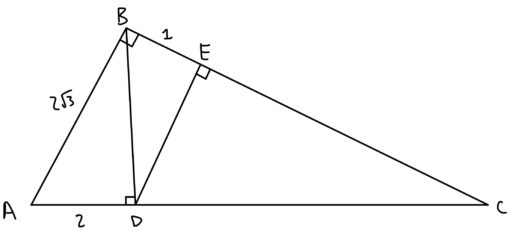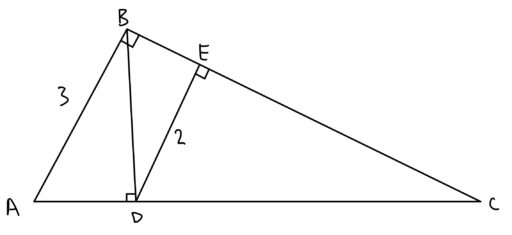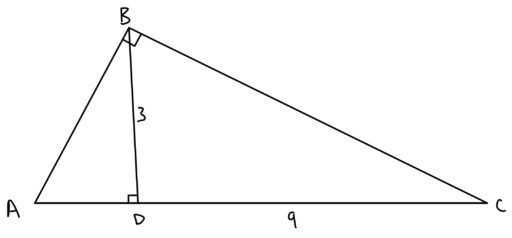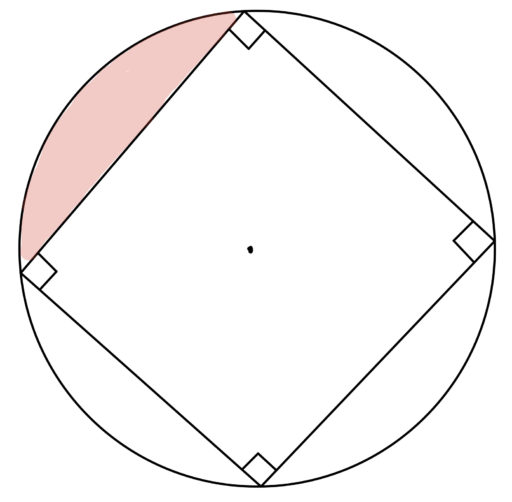Below, we include some additional geometry practice problems.

1. What is the area of? Of?2. In the following diagram,What is the area of?3. What is the area of?4. The radius of the below circle is 3. Find the area of the shaded region.5. The circle below has a radius of. Find the ratio of the shaded region's area to the area of the square below.